# Geometry 2015-2016 SSC (Marathi Semi-English) 10th Standard [इयत्ता १० वी] Question Paper Solution

Geometry
Date: July 2016

1 | Solve any five sub-questions:
1.i

In the following figure RP: PK= 3:2, then find the value of A(ΔTRP):A(ΔTPK).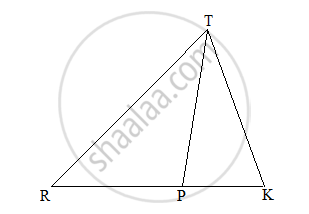Concept: Properties of Ratios of Areas of Two Triangles
Chapter: [0.01] Similarity
1.ii

If two circles with diameters 8 cm and 6 cm respectively touch externally, find the distance between their centers.

Concept: Touching Circles
Chapter: [0.03] Circle
1.iii

Find the Slope of the line having inclination 45°.

Concept: Slope of a Line
Chapter: [0.04] Co-ordinate Geometry
1.iv

Using Euler’s formula, find V if E = 30, F = 12.

Concept: Euler's Formula
Chapter: [0.07] Mensuration
1.v

Find the length of diagonal of the square whose side is 8 cm.

Concept: Right-angled Triangles and Pythagoras Property
Chapter: [0.02] Pythagoras Theorem
1.vi

For the angle in standard position if the initial arm rotates 305° in an anticlockwise direction, then state the quadrant in which the terminal arm lies.

Concept: Angles in Standard Position
Chapter: [0.06] Trigonometry
2 | Solve any four sub-questions:
2.i

Draw seg AB of length 9.7 cm. Take a point P on it such that A-P-B, AP = 3.5 cm. Construct a line MNsag AB through point P.

Concept: Division of a Line Segment
Chapter: [0.04] Co-ordinate Geometry [0.05] Geometric Constructions
2.ii

Find the trigonometric sine ratio of an angle in a standard position whose terminal arm passes through the point (3, 4).

Concept: Trigonometric Ratios in Terms of Coordinates of Point
Chapter: [0.06] Trigonometry
2.iii

In the following figure, state whether the triangles are similar. Give reason.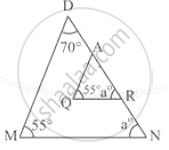Concept: Similar Triangles
Chapter: [0.01] Similarity
2.iv

In the following figure, seg AB is a diameter of the circle, m (arc AKC) = 40°. Find the value of m (arc BMC).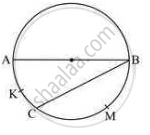Concept: Tangent to a Circle
Chapter: [0.03] Circle
2.v

Write the equation of a line passing through the point P (0,6) and having slope 6/7.

Concept: Slope of a Line
Chapter: [0.04] Co-ordinate Geometry
2.vi

Find the area of sector whose central angle and radius are 60o and 21 cm respectively.
(pi = 22/7)

Concept: Tangent to a Circle
Chapter: [0.03] Circle
3 | Solve any three sub-questions:
3.i

In the following figure, seg AB is the diameter of the circle with center P. Line CB be the tangent and line AC intersects a circle in point D. Prove that: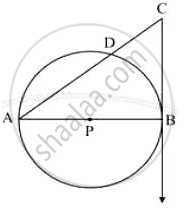Concept: Tangent to a Circle
Chapter: [0.03] Circle
3.ii

In the following figure, ray PT is the bisector of QPR Find the value of x and perimeter of QPR.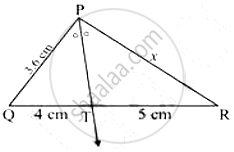Concept: Property of an Angle Bisector of a Triangle
Chapter: [0.01] Similarity
3.iii

The length, breadth, and height of a cuboid are in the ratio 5:4: 2. If the total surface area is 1216 cm2, find dimensions of the solid.

Concept: Surface Area of a Combination of Solids
Chapter: [0.07] Mensuration
3.iv

Construct the incircle of Δ RST in which RS = 6cm, ST 7 cm, RT
= 6.5 cm.

Concept: Inscribed Angle
Chapter: [0.03] Circle
3.v

Show that:

sqrt((1-cos"A")/(1+cos"A"))=cos"ecA - cotA"

Concept: Application of Trigonometry
Chapter: [0.06] Trigonometry
4 | Solve any two sub-questions:
4.i

Prove that ‘the opposite angles of a cyclic quadrilateral are supplementary’.

Chapter: [0.03] Circle
4.ii

A person standing on the bank of river observes that the angle of elevation of the top of a tree standing on the opposite bank is 60°. When he moves 40 m away from the bank, he finds the angle of elevation to be 30°. Find the height of the tree and width of the river. (sqrt 3=1.73)

Concept: Heights and Distances
Chapter: [0.06] Trigonometry
4.iii

A roller of diameter 0.9 m and the length 1.8 m is used to press the ground. Find the area of the ground pressed by it in 500 revolutions.
(pi=3.14)

Concept: Properties of Ratios of Areas of Two Triangles
Chapter: [0.01] Similarity
5 | Solve any two sub-questions:
5.i

Prove that, if a line parallel to a side of a triangle intersects the other sides in two district points, then the line divides those sides in proportion.

Concept: Property of three parallel lines and their transversals
Chapter: [0.01] Similarity
5.ii

Show that ABCD is a parallelogram if A = (4, 8), B = (5, 5), C = (2, 4), D = (1, 7)

Concept: Converse: If a pair of opposite angles of a quadrilateral is supplementary, then the quadrilateral is cyclic.
Chapter: [0.03] Circle
5.iii

ΔAMT∼ΔAHE, construct Δ AMT such that MA = 6.3 cm, ∠MAT=120°, AT = 4.9 cm and "MA"/"HA"=7/5, then construct ΔAHE.

Concept: Application of Trigonometry
Chapter: [0.06] Trigonometry

#### Request Question Paper

If you dont find a question paper, kindly write to us

View All Requests

#### Submit Question Paper

Help us maintain new question papers on Shaalaa.com, so we can continue to help students

only jpg, png and pdf files

## Maharashtra State Board previous year question papers 10th Standard [इयत्ता १० वी] Geometry with solutions 2015 - 2016

Maharashtra State Board 10th Standard [इयत्ता १० वी] Geometry question paper solution is key to score more marks in final exams. Students who have used our past year paper solution have significantly improved in speed and boosted their confidence to solve any question in the examination. Our Maharashtra State Board 10th Standard [इयत्ता १० वी] Geometry question paper 2016 serve as a catalyst to prepare for your Geometry board examination.
Previous year Question paper for Maharashtra State Board 10th Standard [इयत्ता १० वी] Geometry-2016 is solved by experts. Solved question papers gives you the chance to check yourself after your mock test.
By referring the question paper Solutions for Geometry, you can scale your preparation level and work on your weak areas. It will also help the candidates in developing the time-management skills. Practice makes perfect, and there is no better way to practice than to attempt previous year question paper solutions of Maharashtra State Board 10th Standard [इयत्ता १० वी].

How Maharashtra State Board 10th Standard [इयत्ता १० वी] Question Paper solutions Help Students ?
• Question paper solutions for Geometry will helps students to prepare for exam.
• Question paper with answer will boost students confidence in exam time and also give you an idea About the important questions and topics to be prepared for the board exam.
• For finding solution of question papers no need to refer so multiple sources like textbook or guides.